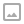## Taking a Look at Groovy @Memoized Functions

·   ·  ☕ 2 min read

Reading up on some of the features of Groovy I stumbled upon the @Memoized annotation. This method annotation allows you to tell Groovy to memoize the results from a function. What is memoizing? Think of it as caching the results of a complex computation.

For example the Fibonacci sequence requires us to recursively calculate f(n) = f(n - 1) + f(n - 2). In order to calculate fibonacci for f(100) this means we need to calculate f(1) a hundred times! Why? The answer is going to be the same every time. The idea of memoizing is to take the results of the complex function and store the results after the first computation into a data structure that makes it easier to lookup. For fibonacci this might be as simple as an array with the results of f(n) stored at index n in our array. The general idea being to replace a complex or slow function with a relatively quick table lookup.

In Groovy we get memoization for free as a feature of the language. By adding @Memoized to a function we can tell Groovy to cache the results of a function for the given inputs and use that cache when the same inputs are used again. In our tests we dropped the time of computing the 40th fibonacci number from over 7 seconds (for 20 computations) to 60 milliseconds. An increase of more than 100 times! And it only took us a single line of code to get this advantage too. That’s a lot of power for a single line of code.

The code from this video is available on GitHub here: https://gist.github.com/runewake2/2d63de01f53619d2d5b976043dc12420

More on Groovy at http://www.groovy-lang.org

The Groovy documentation on @Memoized, including help on managing the backing cache and additional examples: http://docs.groovy-lang.org/latest/html/gapi/groovy/transform/Memoized.html

Wikipedia’s article on Time Complexity and what it means (don’t let some of the more obscure terms intimidate you, focus on constant, logarithmic, linear, exponential and factorial time complexity): https://en.wikipedia.org/wiki/Time_complexity

The World of Zero Discord channel: https://discord.gg/hU5Kq2uWRITTEN BY
Sam Wronski
Maker of things, currently helping build cloud things @ Google. World of Zero is a personal project disconnected from my professional work. Lets make something awesome together!